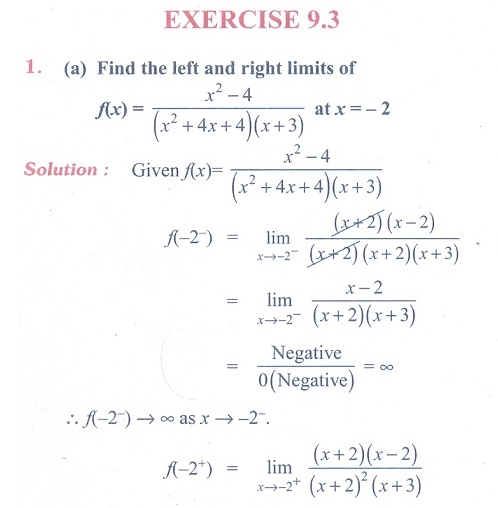Home | | Maths 11th std | Exercise 9.3: Applications of limits

# Exercise 9.3: Applications of limits

Maths Book back answers and solution for Exercise questions - Mathematics : Differential Calculus - Limits and Continuity: Applications of limits

EXERCISE 9.3(9) An important problem in fishery science is to estimate the number of fish presently spawning in streams and use this information to predict the number of mature fish or “recruits” that will return to the rivers during the reproductive period. If S is the number of spawners and R the  number of recruits, “Beverton-Holt spawner recruit function” is R(S) = S / (α S + β ) where α and β are positive constants. Show that this function predicts approximately constant recruitment when the number of spawners is sufficiently large.

(10) A tank contains 5000 litres of pure water. Brine (very salty water) that contains 30 grams of salt per litre of water is pumped into the tank at a rate of 25 litres per minute. The concentration of salt water after t minutes (in grams per litre) is C(t) = 30t / 200+t

What happens to the concentration as t →∞?Tags : Problem Questions with Answer, Solution | Mathematics , 11th Mathematics : UNIT 9 : Differential Calculus Limits and Continuity
Study Material, Lecturing Notes, Assignment, Reference, Wiki description explanation, brief detail
11th Mathematics : UNIT 9 : Differential Calculus Limits and Continuity : Exercise 9.3: Applications of limits | Problem Questions with Answer, Solution | Mathematics